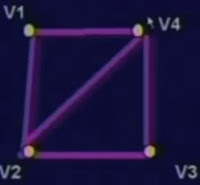# New Technology

Trending Technology Machine Learning, Artificial Intelligent, Block Chain, IoT, DevOps, Data Science## Search This Blog

Instructional Objective
• The students will be introduced to the class of constraint satisfaction problems.
• They will learn how to express different constraint formally.
• They will learn how to model CSP problems as search problems, and how DFS can be used with backtracking to solve these problems.
• They will learn how to cast different CSP problem to search problems.

Introduction

Many problems in AI are types of constraint satisfaction problems.
• Satisfiability
• Scheduling
• Timetabling
• Supply chain management
• Graph colouring
• Machine vision
• Puzzles

Constraint Satisfaction Problems

A CSP consists of :
- a set of variables, X
- for each variable xi in X, a domain Di
• Di is a finite set of possible values
- a set of constraints restricting tuples of values
• if only pairs of values, it's a binary CSP
A solution is an assignment of a value in Di to each variable xj such that every constraints satisfiedColouring as CSP
• Can we colour all 4 regions with 3 colours so  that no two adjacent regions are the same colour?

• Variable for each node- All Di = {red, green, blue}
• Constraint for each edge
- all constraints of the form
xi  ≠ xj
• Solution gives a colouring
• It's a binary CSP

SAT as a CSP
• Variable in CSP for each variable/letter in SAT
• Each domain Di = {true, false}
• Constraint corresponds to each clause

- disallows unique tuple which falsifies clause
- e.g. (not A) or (B) or (not C)
⇒ not < A = true, B = false, C = true >
• Not binary CSP unless all clauses 2-clauses
N-Queens as a CSP
• Chessboard puzzle
- place 8 queens on a 8x8 chessboard so that no two attack each other
• Variable xi for each row i of the board
• Domain = {1,2,3.....,n} for position in row

Formal Definition of Constraints

A constraint Cijk.... involving variables xi, xj, xk ...
- is any subset of combination of values from Di, Dj, Dk ....
- i.e. Cijk ... ⊆ Di x Dj x Dk...
- indicating the allowed set of values
A number of ways to write constraints:
- e.g. if D1 - D2 = {1,2,3} ...
•     { (1,2), (1,3), (2,1), (2,3),(3,1),(3,2)}
• x1 ≠ x2
More Complex Constraints